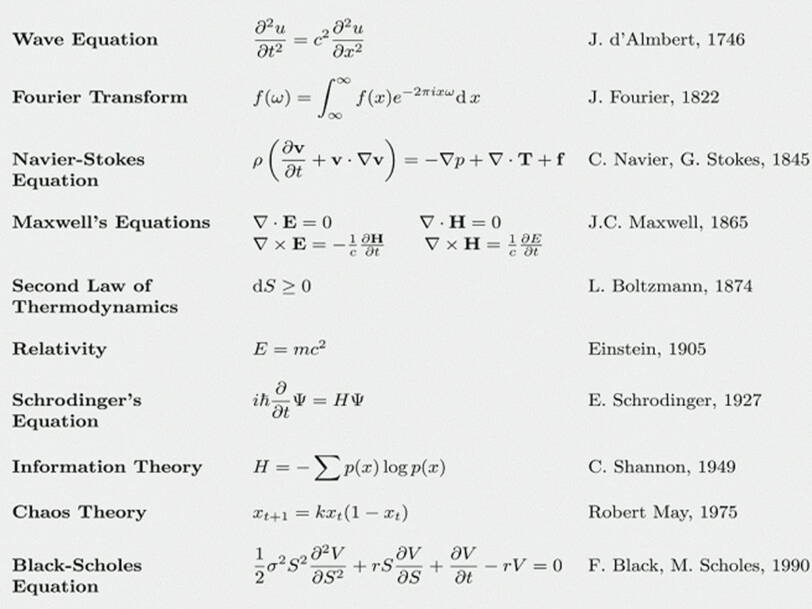#From Ian Stewart's book, these 17 math equations changed the course of human history

• A 2013 book by mathematician and science author Ian Stewart looked at 17 mathematical equations that shaped our understanding of the world.
• From basic geometry to our understanding of how the physical world works to the theories underlying the internet and our financial systems, these equations have changed human history.

Mathematics is all around us, and it has shaped our understanding of the world in countless ways.

In 2013, mathematician and science author Ian Stewart published a book on "17 Equations That Changed The World."Views: 2619

Comment

Join Data Science CentralComment by Pablo Gutierrez on April 16, 2020 at 9:50am

Very nice post!

I belive that the Shanon equation for information theory is related to the definition of Entropy and its nature, so equations 6 and 9 would be connected.